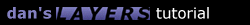Although a circle is a simple shape, moving a layer in a circular path is rather complicated. I've taken great care so that you don't have to bother with any of the math.

I created a separate function where I set all the options for the circle. The lines with descriptions after the "//" are the ones that you can change. I've found this technique works very well, and I'll continue with it for all of the pre-built functions.

When calling the function you just call "circle()" to set the options you want and then it will automatically call the function "slidecircle()" which does all the mathematical work. I am using global variables here so to make sure that variables in other functions don't interfere with eachother I've named each one starting with "Circ". Most of the options should be self-explanitory - the CircVertical value can be used to make it into an oval.

```function circle() {
CircLayername = "layer1";  // name of the layer you want to move
CircVertical = 1.0;        // vertical multiplier, <1 makes thinner, >1 makes fatter
CircHorizontal = 1.0;      // horizontal multiplier
CircAnglestart = 0;        // starting angle (0 is the right edge of the circle, 90 is at the top and so on)
CircAngleinc = 5;          // angle incremenation, a larger number makes it go faster
CircCycles = 1.0;          // the number of times you want to go around (decimals allowed)
CircDirection = 1;         // "1" is counter-clockwise, "-1" is clockwise
CircSpeed = 30;            // repetition speed in milliseconds
CircAngle = CircAnglestart;
slidecircle();
}

function slidecircle() {
if (Math.abs(CircAngle - CircAnglestart) < CircCycles*360) {
CircAngle += CircDirection*CircAngleinc;
var x = CircLeft + CircHorizontal*CircRadius*Math.cos(CircAngle*Math.PI/180);
var y = CircTop - CircVertical*CircRadius*Math.sin(CircAngle*Math.PI/180);
document.layers[CircLayername].moveTo(x,y);
setTimeout("slidecircle()",CircSpeed);
}
}
```

If you want the function to loop indefinitely you can remove the "if" statement entirely.1.1 Introduction 1.2 Overlapping 1.3 Nesting 1.4 Using JavaScript 2.1 Sliding Layers 2.2 Pre-Built Functions 2.3 Clipping Layers 2.4 Looping Animations 2.5 Changing Images 3.1 Mouse-Click Animation 3.2 Capturing Keystrokes 3.3 Drag and Drop 4.1 Making Demos 4.4 Problems 4.5 Screen Sizes Copyright © 1997 Dan Steinman. All rights reserved.